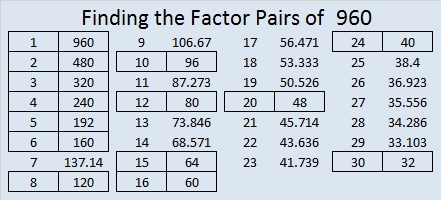960 Factor Trees

Contents

Factor Trees for 960:

960 is the smallest number to have exactly 28 factors. 960 is 2⁶·3·5, so any factor tree made for it will have 6 + 1 + 1 = 8 prime factors. Since 8 is a power of 2, this number, 960, has some beautiful and well-balanced factor trees as well as some that aren’t as good-looking. Here are five of the MANY possible factor trees for 960:Factors of 960:

• 960 is a composite number.
• Prime factorization: 960 = 2 × 2 × 2 × 2 × 2 × 2 × 3 × 5, which can be written 960 = 2⁶ × 3 × 5
• The exponents in the prime factorization are 6, 1 and 1. Adding one to each and multiplying we get (6 + 1)(1 + 1)(1 + 1) = 7 × 2 × 2 = 28. Therefore 960 has exactly 28 factors.
• Factors of 960: 1, 2, 3, 4, 5, 6, 8, 10, 12, 15, 16, 20, 24, 30, 32, 40, 48, 60, 64, 80, 96, 120, 160, 192, 240, 320, 480, 960
• Factor pairs: 960 = 1 × 960, 2 × 480, 3 × 320, 4 × 240, 5 × 192, 6 × 160, 8 × 120, 10 × 96, 12 × 80, 15 × 64, 16 × 60, 20 × 48, 24 × 40, or 30 × 32
• Taking the factor pair with the largest square number factor, we get √960 = (√64)(√15) = 8√15 ≈ 30.9838668Sum-Difference Puzzles:

60 has six factor pairs. One of those pairs adds up to 17, and  another one subtracts to 17. Put the factors in the appropriate boxes in the first puzzle.

960 has fourteen factor pairs. One of the factor pairs adds up to ­68, and a different one subtracts to 68. If you can identify those factor pairs, then you can solve the second puzzle!The second puzzle is really just the first puzzle in disguise. Why would I say that?

960 can be written as the difference of 2 squares TEN different ways:

1. 241² – 239² = (241 + 239)(241 – 239) = 480 × 2 = 960
2. 122² – 118² = (122 + 118)(122 – 118) = 240 × 4 = 960
3. 83² – 77² = (83 + 77)(83 – 77) = 160 × 6 = 960
4. 64² – 56² = (64 + 56)(64 – 56) = 120 × 8 = 960
5. 53² – 43² = (53 + 43)(53 – 43) = 96 × 10 = 960
6. 46² – 34² = (46 + 34)(46 – 34) = 80 × 12 = 960
7. 38² – 22² = (38 + 22)(38 – 22) = 60 × 16 = 960
8. 34² – 14² = (34 + 14)(34 – 14) = 48 × 20 = 960
9. 32² – 8² = (32 + 8)(32 – 8) = 40 × 24 = 960
10. 31² – 1² = (31 + 1)(31 – 1) = 32 × 30 = 960

960 is the sum of the sixteen prime numbers from 29 to 97.

It is also the sum of six consecutive prime numbers:
149 + 151 + 157 + 163 + 167 + 173 = 960

960 is the hypotenuse of a Pythagorean triple:
576-768-960 which is (3-4-5) times 192

I like how 960 looks in these other bases:
33000 in BASE 4 because 3(4⁴) + 3(4³) = 3(256 + 64) = 3 × 320 = 960
440 in BASE 15 because 4(15²) + 4(15) = 4(225 + 15) = 4 × 240 = 960
UU in BASE 31 (U is 30 base 10), because 30(31) + 30(1) = 30(31 + 1) = 30 × 32 = 960
U0 in BASE 32 because 30(32) + 0 = 960

OEIS.org informs us that 9 + 6 + 09³ + 6³ + 0³ = 960

This site uses Akismet to reduce spam. Learn how your comment data is processed.